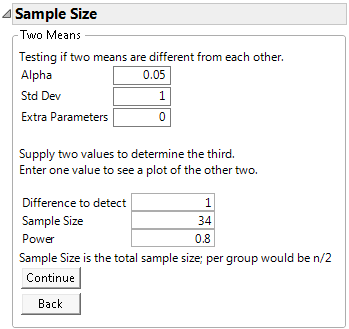Publication date: 08/13/2020

## Example of the Two Sample Means Calculator

Suppose you are comparing two groups and want to detect a 1 standard deviation difference between the group means at a 0.05 significance level with 80% power.

1. Select DOE > Design Diagnostics > Sample Size and Power.

2. Click Two Sample Means.

3. Leave Alpha as 0.05.

4. Enter 1 for Std Dev.

5. Leave Extra Parameters as 0.

6. Enter 1 as Difference to detect.

7. Leave Sample Size blank.

8. Enter 0.8 for Power.

9. Click Continue.

Figure 17.8 Two Sample Means Calculator ResultThe sample size is calculated as 34. With 17 samples in each group, you have an 80% chance of detecting a 1 standard deviation difference between the two sample means at a significance level of α = 0.05.